# JavaScript drawing - Exercises, Practice, Solution

## JavaScript drawing [6 exercises with solution]

[An editor is available at the bottom of the page to write and execute the scripts.]

1. Write a JavaScript program to draw the following rectangular shape. Go to the editor
Expected Output :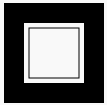Click me to see the solution

2. Write a JavaScript program to draw a circle. Go to the editor
Expected Output :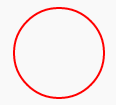Click me to see the solution

3. Write a JavaScript program to draw two intersecting rectangles, one of which has alpha transparency. Go to the editor
Expected Output :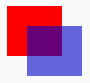Click me to see the solution

4. Write a JavaScript program to draw the following right-angled triangle. Go to the editor
Expected Output :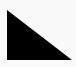Click me to see the solution

5. Write a JavaScript program to draw the following diagram [use moveto() function]. Go to the editor
Expected Output :Click me to see the solution

6. Write a JavaScript program to draw the following diagram [diagonal, white to black circles]. Go to the editor
Expected Output :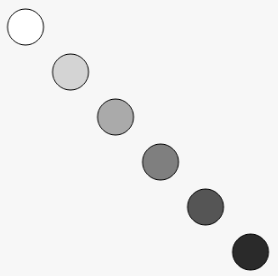Click me to see the solution

More to Come !

* To run the code mouse over on Result panel and click on 'RERUN' button.*

Live Demo:

See the Pen javascript-common-editor by w3resource (@w3resource) on CodePen.

﻿

## JavaScript: Tips of the Day

Applies a function against an accumulator and each key in the object (from left to right)

Example:

```const transform = (obj, fn, acc) => Object.keys(obj).reduce((a, k) => fn(a, obj[k], k, obj), acc);
console.log(transform(
{ a: 1, b: 2, c: 1 },
(r, v, k) => {
(r[v] || (r[v] = [])).push(k);
return r;
},
{}
)); // { '1': ['a', 'c'], '2': ['b'] }
```

Output:

```[object Object] {
1: ["a", "c"],
2: ["b"]
}
```